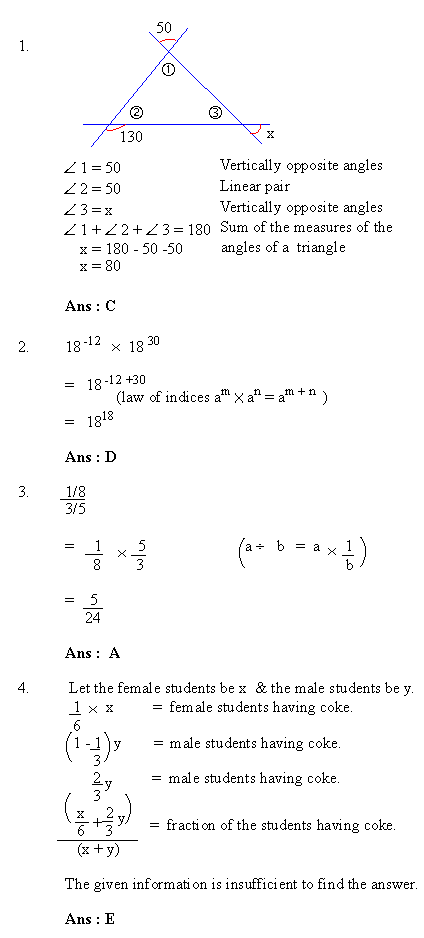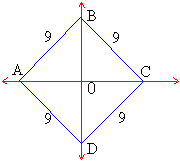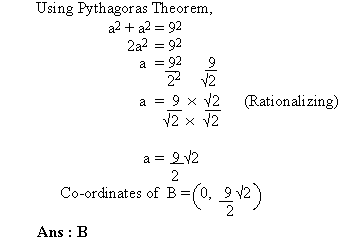Support the Monkey! Tell All your Friends and TeachersHome MonkeyNotes Printable Notes Digital Library Study Guides Study Smart Parents Tips College Planning Test Prep Fun Zone Help / FAQ How to Cite New Title Request

 Section 2 : Mathematical ReasoningLet the edge of the cube be x.       Volume of the cube = x3        x3 = 125       x = 5 inches.       Number of inches of wire used to form the cube       = 12x       = 12 ´ 5       = 60 inches.       Ans : A      6.       Paul's age 5 years ago was x years.             \ Present age of Paul = (x + 5) years             From the given information,               Wilson is one-fourth of Paul's age now.       = 1 (x + 5) years4       Ans : B      7.      Let the side of the square be x.Area of square = x2       Since B is a point on the y- axis. Its x - co-ordinate is 0.       Co-ordinates of point B º (0,a) where a has to be calculated.       To find side of a square, we solve x2 = 81, i. e. x = ± 9       Diagonals of a square are equal and they are perpendicular bisectors of each other.        Let  l(OB) = l(OA) = aIndex Test 1 Section 1 : Verbal Reasoning Section 2 : Mathematical Reasoning Section 3 : Verbal Reasoning Section 4 : Mathematical Reasoning Section 5 : Reading Comprehension Section 6 : Mathematical Reasoning Section 7 : Mathematical Reasoning Answer Key To Test 1 Answer Explanation To Test 1 Section 1 : Verbal Reasoning Section 2 : Mathematical Reasoning Section 3 : Verbal Reasoning Section 4 : Mathematical Reasoning Section 5 : Reading Comprehension Section 6 : Mathematical Reasoning Section 7 : Mathematical Reasoning Test 2
 Search: All Products Books Popular Music Classical Music Video DVD Toys & Games Electronics Software Tools & Hardware Outdoor Living Kitchen & Housewares Camera & Photo Cell Phones Keywords: